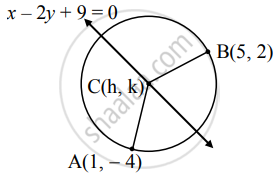Advertisement Remove all ads

# Find the equation of a circle passing through the points (1,−4), (5,2) and having its centre on the line x − 2y + 9 = 0 - Mathematics and Statistics

Sum

Find the equation of a circle passing through the points (1,−4), (5,2) and having its centre on the line x − 2y + 9 = 0

Advertisement Remove all ads

#### SolutionLet C(h, k) be the centre of the required circle

which lies on the line x – 2y + 9 = 0.

∴ Equation of line becomes

h – 2k + 9 = 0 …(i)

Also, the required circle passes through the points A(1, – 4) and B(5, 2).

∴ CA = CB = radius

CA = CB

By distance formula,

sqrt(("h" - 1)^2 + ["k" - (- 4)]^2) =  sqrt(("h" - 5)^2 + ("k" - 2)^2)

Squaring both the sides, we get

(h – 1)2 + (k + 4)2 = (h – 5)2 + (k – 2)2

∴ h2 – 2h + 1 + k2 + 8k + 16 = h2 – 10h + 25 + k2 – 4k + 4

∴ – 2h + 8k + 17 = – 10h – 4k + 29

∴ 8h + 12k – 12 = 0

∴ 2h + 3k – 3 = 0 …(ii)

By (ii) – (i) x 2, we get

7k = 21

∴ k = 3

Substituting k = 3 in (i), we get

h – 2(3) + 9 = 0

∴ h – 6 + 9 = 0

∴ h = – 3

∴ Centre of the circle is C (– 3, 3).

radius (r) = CA

= sqrt([1 - (-3)]^2 + (-4 - 3)^2)

= sqrt(4^2 + (-7)^2)

= sqrt(16 + 49)

= sqrt(65)

The equation of a circle with centre at (h, k) and radius r is given by

(x – h)2 + (y – k)2 = r2

Here, h = – 3, k = 3, r = sqrt(65)

∴ The required equation of the circle is

[x – (–3)]2 + (y – 3)2 = (sqrt(65))^2

∴ (x + 3)2 + (y – 3)2 = 65

∴ x2 + 6x + 9 + y2 – 6y + 9 – 65 = 0

∴ x2 + y2 + 6x – 6y – 47 = 0.

Is there an error in this question or solution?
Advertisement Remove all ads

#### APPEARS IN

Advertisement Remove all ads
Advertisement Remove all ads
Share
Notifications

View all notifications

Forgot password?